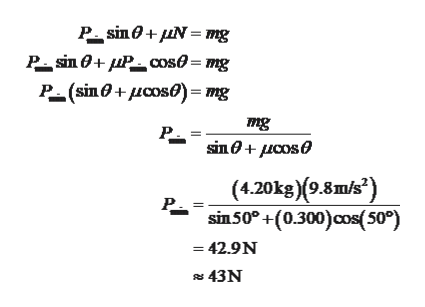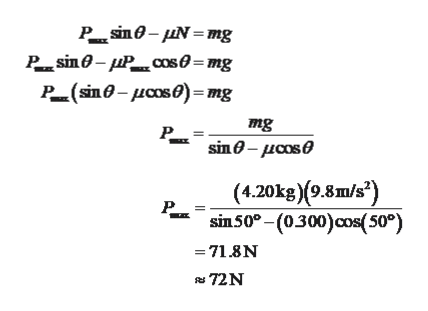# A block of mass 4.20 kg is pushed up against a wall by a force P that makes an angle of θ = 50.0° angle with the horizontal as shown below. The coefficient of static friction between the block and the wall is 0.300. (a) Determine the possible values for the magnitude of P that allow the block to remain stationary. (If there is no maximum, enter NONE in that answer blank.)|Pmax|=  N|Pmin|=  N

Question
5 views

A block of mass 4.20 kg is pushed up against a wall by a force P that makes an angle of θ = 50.0° angle with the horizontal as shown below. The coefficient of static friction between the block and the wall is 0.300.

(a) Determine the possible values for the magnitude of P that allow the block to remain stationary. (If there is no maximum, enter NONE in that answer blank.)
 |Pmax| =  N |Pmin| =  N
check_circle

Step 1

(a)

The minimum force exerted on the block to avoid slide and the friction force will acts upwards.

Substitute the values,help_outlineImage TranscriptioncloseP sin+Nmg Р яiл 0+ пP_ соs0 - тg Р- (sin @ + дсоsе)- тg = mg sin@ + дсose (4.20kg)(9.8m/s2) sin50° +(0.300)cos(50°) Р. =42.9N 43N fullscreen
Step 2

The maximum force exerted on the block to sliding upwards and the fr...help_outlineImage TranscriptioncloseP.sn0- μΝ =m P.sinθ-μP.. cs0- mg P(nθ- μοs 0) = mg πag Р. sin θ- μαs θ (4.20ks) (9.3π/s") Р. sin50°-(0300)cos(50°) -71.8N 72N fullscreen

### Want to see the full answer?

See Solution

#### Want to see this answer and more?

Solutions are written by subject experts who are available 24/7. Questions are typically answered within 1 hour.*

See Solution
*Response times may vary by subject and question.
Tagged in

### Newtons Laws of Motion